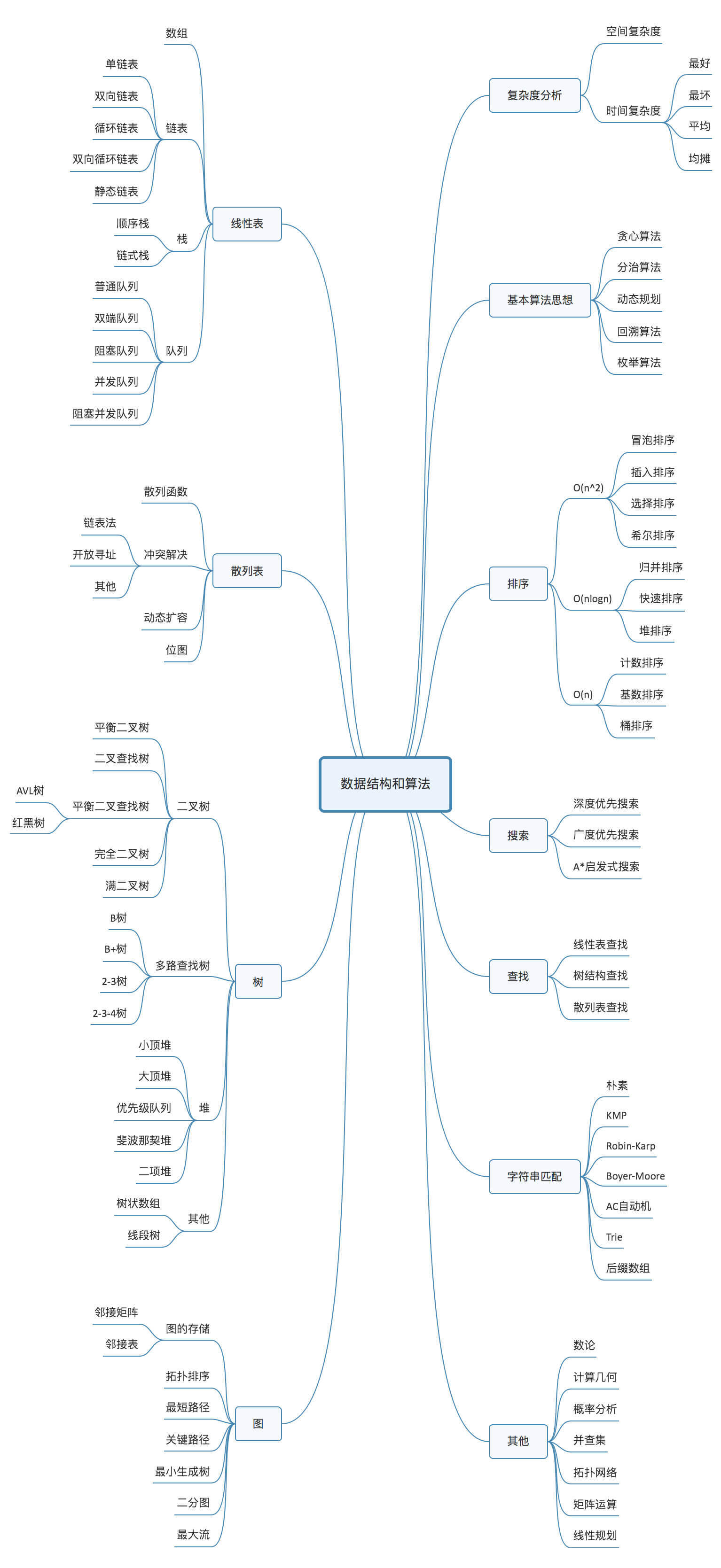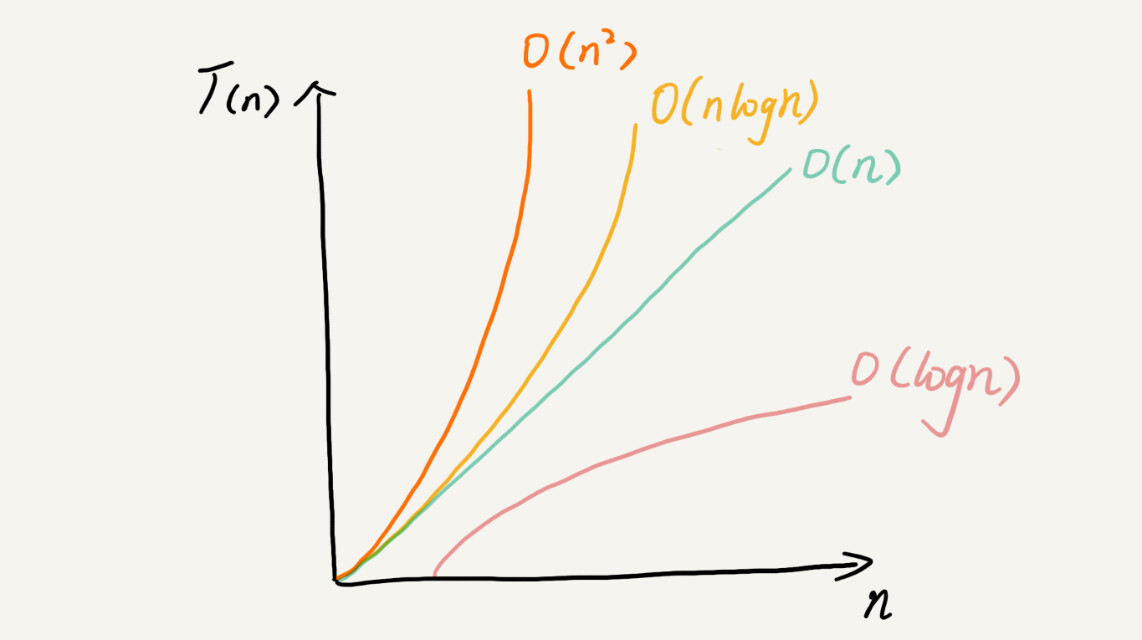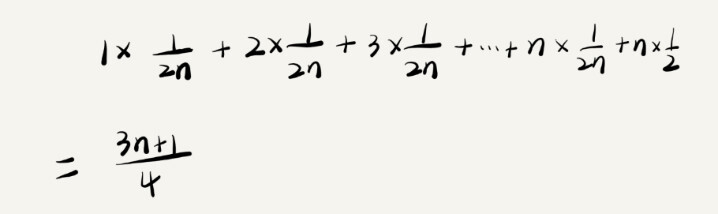# 数据结构与算法之美学习笔记

## 入门篇

Posted by Alee on September 10, 2019

## 入门篇

#### 数据结构和算法所有知识点图谱#### 20个最常用的、最基础的数据结构与算法

10个数据结构：数组、链表、栈、队列、散列表、二叉树、堆、跳表、图、Trie树；

10个算法：递归、排序、二分查找、搜索、哈希算法、贪心算法、分治算法、回溯算法、动态规划、字符串匹配算法；

#### 算法学习技巧

1. 边学边练，适度刷题
2. 多问、多思考、多互动
3. 打怪升级学习法
4. 知识需要沉淀，不要想试图一下子掌握所有

#### 为什么需要复杂度分析？

1. 测试结果非常依赖测试环境
2. 测试结果受数据规模的影响很大

#### 大O复杂度表示法

T(n) = O(f(n))
T(n)表示代码执行的时间
n表示数据规模的大小
f(n)表示每行代码执行的次数总和，因为这是一个公式，所以用f(n)来表示



#### 时间复杂度分析

1. 只关注循环执行次数最多的一段代码
2. 加法法则：总复杂度等于量级最大的那段代码的复杂度
3. 乘法法则：嵌套代码的复杂度等于嵌套内外代码复杂度的乘积
int cal(int n) { //举个例子
int ret = 0;
int i = 1;
for(: i < n: ++i) {
ret = ret + f(i);
}
return ret;
}

int f(int n) {
int sum = 0;
int i = 1;
for (;  i < n; ++i) {
sum = sum + i;
}
return sum;
}



#### 几种常见时间复杂度实例分析

• 常量阶 O(1)
• 对数阶 O(㏒n)
• 线性阶 O(n)
• 线性对数阶 O(n㏒n)
• 方法阶 O(n²)
• 立方阶 O(n³)
• K次方阶 O(nᴷ)
• 指数阶 O(2ⁿ)
• 阶乘阶 O(n!)

1. O(1)

表示一种常量级时间复杂度，并不是指只执行了一行代码。一般情况下，只要算法中不存在循环语句、递归语句，即使有成千上万行的代码，其时间复杂度也是O(1)。

2. O(㏒n)、O(n㏒n)

对数阶时间复杂度非常常见，同时也是最难分析的一种时间复杂度。

int i = 1;
while (i <= n) {
i = i * 2;
}
从代码中可以看出，变量 i 的值从 1 开始取，每循环一次就乘以 2。当大于 n 时，循环结束。
还记得我们高中学过的等比数列吗？实际上，变量 i 的取值就是一个等比数列。
如果我把它一个一个列出来，就应该是这个样子的：
2⁰ 2¹ 2² 2³ ... 2ᵏ ...2ˣ = n
所以，我们只要知道 x 值是多少，就知道这行代码执行的次数了。
通过 2ˣ=n 求解 x 这个问题我们想高中应该就学过了，我就不多说了。
x=㏒₂n，所以，这段代码的时间复杂度就是 O(㏒₂n)

int i = 1;
while (i <= n) {
i = i * 3;
}
时间复杂度为O(㏒₃n)
实际上，不管是以 2 为底、以 3 为底，还是以 10 为底，我们忽略对数的“底”，
可以把所有对数阶的时间复杂度都记为 O(㏒n)。


O(n㏒n)就是把一段时间复杂度是O(㏒n)的代码循环执行n遍的时间复杂度，O(n㏒n)也是一种非常常见的算法时间复杂度。比如，归并排序、快速排序。

3. O(m+n)、O(m*n)

int cal(int m, int n) {
int sum_1 = 0;
int i = 1;
for (; i < m; ++i) {
sum_1 = sum_1 + i;
}
int sum_2 = 0;
int j = 1;
for (; j < n; ++j) {
sum_2 = sum_2 + j;
}
return sum_1+sum_2;
}
从代码中可以看出，m 和 n 是表示两个数据规模。我们无法事先评估 m 和 n 谁的量级大，
所以我们在表示复杂度的时候，就不能简单地利用加法法则，省略掉其中一个。
所以，上面代码的时间复杂度就是 O(m+n)。


针对这种情况，原来的加法法则就不正确了，我们需要将加法规则改为：T1(m) + T2(n) = O(f(m) + g(n))。但是乘法法则继续有效：T1(m)*T2(n) = O(f(m) * f(n))。

#### 空间复杂度分析

void print(int n) {
int i = 0;
int[] a = new int[n];
for (; i < n; i++) {
a[i] = i * i;
}
for (i = n - 1; i >= 0; --i) {
print out a[i];
}
}



#### 阶段小结#### 最好、最坏情况时间复杂度

// n 表示数组 array 的长度
int find(int[] array, int n, int x) {
int i = 0;
int pos = -1;
for (; i < n; ++i) {
if (array[i] == x) pos = i;
}
return pos;
}



// n 表示数组 array 的长度
int find(int[] array, int n, int x) {
int i = 0;
int pos = -1;
for (; i < n; ++i) {
if (array[i] == x) {
pos = i;
break;
}
}
return pos;
}



#### 平均情况时间复杂度$1\times \frac{1}{2n}+2\times \frac{1}{2n}+3\times \frac{1}{2n}+...+n\times \frac{1}{2n}+n\times \frac{1}{2}=\frac{3n+1}{4}$ 这个值就是概率论中的加权平均估值，也叫做期望值，所以平均时间复杂度的全称应该叫加权平均时间复杂度期望时间复杂度

#### 均摊时间复杂度

 // array 表示一个长度为 n 的数组
// 代码中的 array.length 就等于 n
int[] array = new int[n];
int count = 0;

void insert(int val) {
if (count == array.length) {
int sum = 0;
for (int i = 0; i < array.length; ++i) {
sum = sum + array[i];
}
array = sum;
count = 1;
}

array[count] = val;
++count;
}#### 思考题

// 全局变量，大小为 10 的数组 array，长度 len，下标 i。
int array[] = new int;
int len = 10;
int i = 0;

// 往数组中添加一个元素
if (i >= len) { // 数组空间不够了
// 重新申请一个 2 倍大小的数组空间
int new_array[] = new int[len*2];
// 把原来 array 数组中的数据依次 copy 到 new_array
for (int j = 0; j < len; ++j) {
new_array[j] = array[j];
}
// new_array 复制给 array，array 现在大小就是 2 倍 len 了
array = new_array;
len = 2 * len;
}
// 将 element 放到下标为 i 的位置，下标 i 加一
array[i] = element;
++i;
}


1. 最好的情况是O(1)，最坏的情况是O(n)，大部分情况是O(1)，则均摊是O(1)